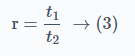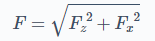Search
•Abhi Shejul

# Merchant's circle and cutting force analysis

Merchant’s circle is used for establishing relationship between measurable and actual forces!

### Table of Content

Short VideoEarlier we had seen the process of chip formation and what are the different types of chips formed during the process of machining or cutting. The various angles on the cutting tool has a significant contribution on the size and shape of the chips formed. But, along with this, the forces involved in any machining process contributes to the size and shape (thickness) of the chip or vice versa.

Today we are going to take a look at what are these forces are and how to calculate these forces.

First, lets take a look at the orthogonal cutting process. In orthogonal cutting process, a single point cutting tool is provided with FEED towards the rotating workpiece. Therefore the cutting force has two components, One in the feed direction (Fd) and other in direction of cutting (Fc).

The cutting tool moves in the direction of the feed. Along the shear plane, the metal deforms plastically. The chips roll along the tool's rake face. The roughness of the chip causes movement resistance, resulting in a frictional force F acting on the chip.

## Types of Forces

So, various forces acting are:

Force F:

Frictional resistance of the tool acting on the chip.

Force N:

Reaction provided by the tool, acting in a direction normal to the rake face of the tool. It is normal to frictional force F.

Force Fs:

Shear force of the metal. It is due to resistance of the metal to shear in forming the chips.

Force Fn:

Normal force to shear plane. It is a backing up force provided by the workpiece on the chip. It causes compressive stress on the shear plane.

Now that we have understood the forces involved, how do we determine these forces?Orthogonal Cutting

The best way to get around the analysis of these forces is via Merchant circle diagram.

This analysis is based on the Merchant equation. The Merchant equation states that

The shear plane angle increases when rake angle is increased and friction angle is decreased.

This means that the forces along these forces will be impacted by the various angles. Each analysis is a triangle of forces.

## Assumptions

What are the assumptions made when we draw the Merchant Circle Diagram?

• A sharp cutting instrument is required.

• The cutting tool does not make contact with the clearance edge.

• There is no built-up edge and the chip creation is continuous.

• The uncut chip has a consistent thickness.

• During the cutting operation, the workpiece travels at a constant speed.

• The workpiece width is always smaller than the cutting tool width.

• Shear occurs in a shear plane with homogenous stress distribution.Cutting tool - Chip - Force diagram

## Triangle of Forces

The three triangles of forces in merchant’s circle diagram are

Cutting forces,

Shear forces,

Frictional forces.

Let's take a look at how the Merchant's Force analysis diagram is drawn. The direction in which the forces are acting are the tool angle and friction angle.

### Cutting forces triangle:

• The cutting force is acting along the direction of the tool.

• The thrust force is perpendicular to the cutting force i.e. in the direction of feed into the workpiece.

• The resultant of the cutting force and thrust force is the resultant force. This forms the diameter of the merchants circle diagram.

### Frictional forces triangle:

• The friction force is acting along the direction of the chip flow, i.e. at the rake angle.

• The normal force is perpendicular to the friction force and meets the thrust force at a common point.

• The resultant of the friction force and normal force is the resultant force and is same as the one we saw earlier.

### Shear forces triangle:

• The shear force is acting along the cross section of the chip which is formed. Hence, the shear force is drawn at the shear angle and has length of the thickness of the chip.

• The normal shear force is perpendicular to the shear force.

• The resultant of the shear force and normal shear force is the resultant force and is same as the one we saw earlier.

In the three triangles we have seen above, the resultant force is same and this resultant force is the diameter of the Merchant's circle. A dynamometer is used to analyse the cutting force and thrust force mentioned above. These forces are then analysed and represented on a suitable scale.

## Force RelationshipsFrom the above diagram, the chip thickness ratio is denoted as r.where,(t1 and t2 are thickness of chip before and after cutting)

Therefore,We know that cos(A-B) = cosAcosB + sinAsinBFrom the Merchant's diagram we know that,The coefficient of friction will be,By trigonometric relations, the value of Fs and Fn is obtained from the merchant circle diagram.## Drawing the Merchant's Circle Diagram

Consider the state of an orthogonal cutting process with the specified feed, tool geometry, and cut depth. We now only have the values of two forces, which are measured with a dynamometer. We will derive the other force relations quickly and easily using the merchant's circle diagram and associated equations.

Chip and tool are drawn on the orthogonal plane at the rake angle of the tool.

Identify the Thrust Force (Fx) and draw the horizontal force as thrust force, and vertical force (Fz) as the cutting force.

Draw a resultant for both these forces Fx and Fy asConsidering the resultant Force (F) as diameter, draw a circle from the centre of the resultant force (F).

Extending the rake surface of the tool, draw a line meeting the circle. This will represent the Friction force (P).

Draw a perpendicular to this friction force, which meets at the resultant force. This is normal force. (N)

The coefficient of friction is calculated using the formula:At the point of contact of tool and workpiece, draw the shear plane at the shear angle.

Draw the Shear Force (Fs) and Normal Shear force (Fns) at this shear plane. Shear force will be along the shear plane and Normal Shear force is perpendicular to the shear force at inception.

## Summary:

Merchant's circle diagram establishes a relationship between the actual forces and measurable forces in the cutting process.

There are three force systems which can be analysed. Cutting, shear and friction.

The resultant force of all these systems is same. Which is mapped as the diameter of the merchant's circle.

Friction at the tool surface and yield shear strength can both be easily evaluated using Merchant's Circle diagram.

## Short Video:

So next time you are doing any cutting force analysis, be sure to check the Merchant's equation and use the Merchant Circle Diagram. And for more fun content related to Mechanical engineering, be sure to follow Mech n Flow.